# K vs. Coq as Language Verification Frameworks (Part 3 of 3)

Posted on December 12, 2019 by

Posted in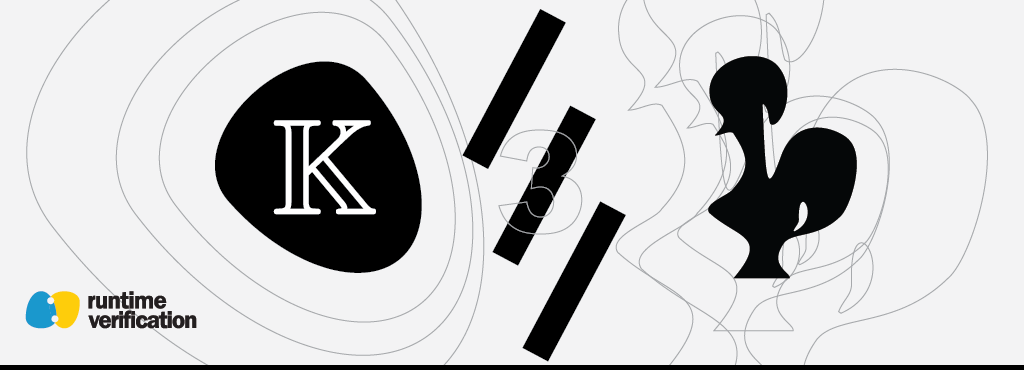Musab A. Alturki and Brandon Moore

This is the third and last part of the “K vs. Coq as Language Verification Frameworks” post series. After illustrating how the syntactic structures of a language are defined (Part 1), and how its semantics can be defined and tested (Part 2) in both K and Coq, we now describe how the third step in the verification workflow, which is specifying and verifying correctness properties, can be achieved. We use the same working example SUM and assume all the language definitions of IMP and SUM described in the previous parts.

Recall that the correctness property we would like to verify is that the program SUM indeed computes the summation of values from 1 through n, with n being a non-negative integer. We show the program SUM here again for easy reference: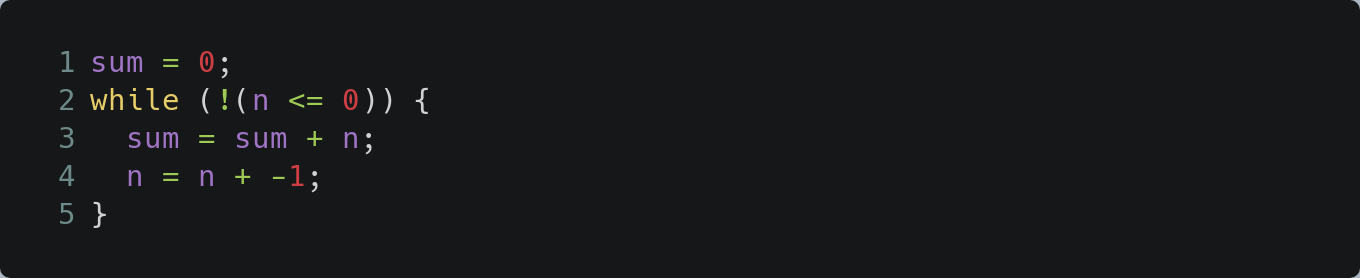Our working example program SUM

## Specifying the Correctness Property of SUM

Before verifying the property, we first need to specify precisely what the property states.

### Property specification in K

Correctness properties about programs in a language defined in K can be specified as reachability rules in reachability logic of the form `p /\ c => p`, with `p` and `p’` patterns in matching logic and `c` a side condition. Intuitively, the assertion states that an instance of `p` (a configuration) for which `c` is satisfied reaches an instance of `p’` along some path (for one-path reachability) or along all paths (for all-path reachability) of execution (see this paper for more precise definitions). Note that the same K construct, namely the K rule, is used uniformly for both defining language semantics and stating reachability properties.

Since IMP is a deterministic language (i.e., any IMP program can have only one possible execution), one-path and all-path reachability coincide.

In particular, when specifying the correctness property of `SUM` as the reachability rule `p /\ c => p` :

• `p` is a pattern that specifies initial configurations of `SUM` in which the `<k>` cell has the entire program sum to be executed and the `<state>` cell is empty.
• `p’` is a pattern that specifies final configurations in which the `<k>` cell is empty (the program completed its execution) and the `<state>` cell maps `n` to 0 and `sum` to the value `(n + 1) n / 2` (i.e. `sum` has the expected value).
• `c` is the condition that the initial value of `n` is non-negative.

In K, this reachability rule is specified using the same syntactic definitions and semantic structures defined in the K model of IMP. The rule specifying the correctness property is shown below (the `requires` clause specifies the side condition):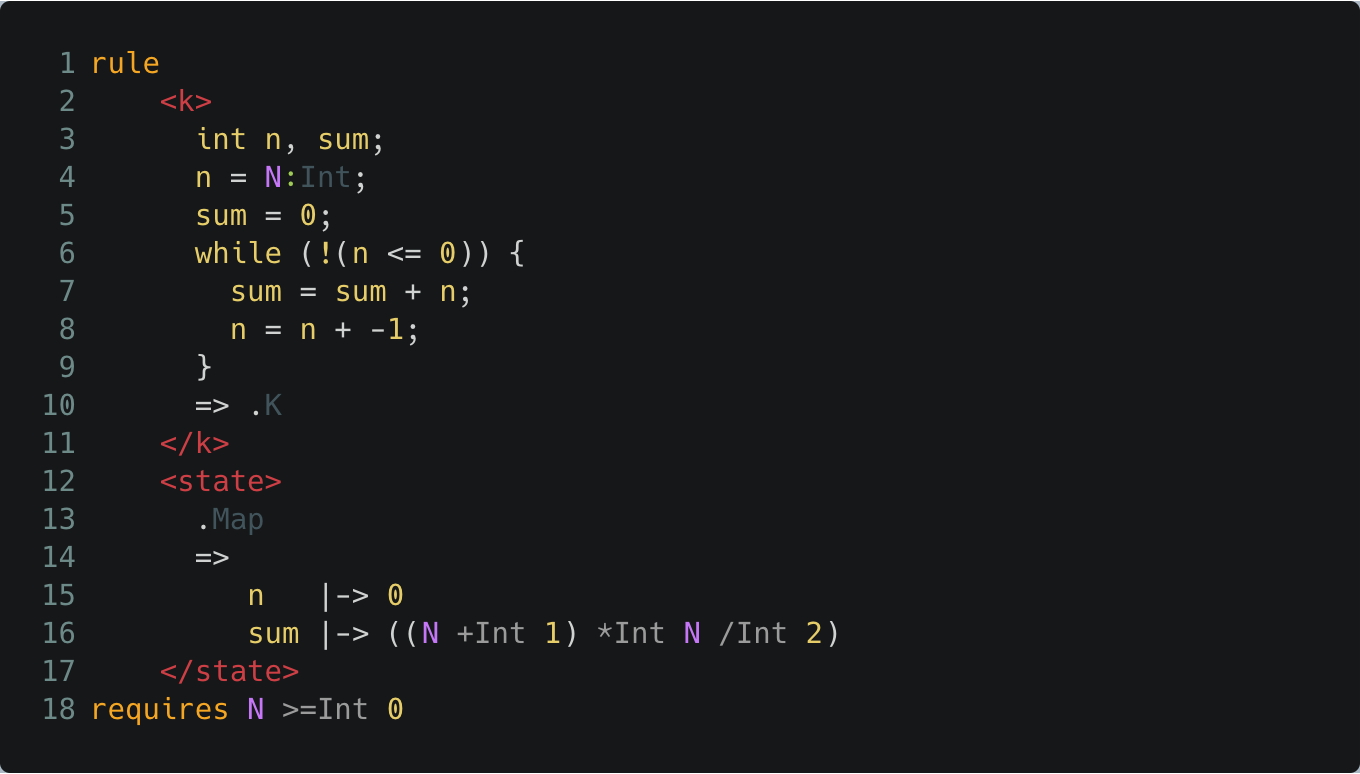The correctness property of SUM in K

Note that the specification is symbolic: the program variable `n` is assigned a symbolic integer value `N`, which is constrained by the rule to be non-negative. The rule specifies that when the program terminates (the cell is the empty computation), the state maps `sum` to the value of the symbolic expression given in terms of `N`, the initial value of the variable `n`.

While the rule above defines the main correctness property of SUM in K, other more specific properties about the language and/or the program may also need to be defined and proved as lemmas before a full proof of the main property can be developed (which is typical in deductive verification methods).

For example, for SUM, a loop invariant specifying how the while loop updates the program variables in every iteration of the loop will also need to be specified (and proved). We elaborate further on the verification process in an upcoming section, but the point to keep in mind here is that all properties are specified as K rules similar in structure to the rule above.

### Property Specification in Coq

To reason about IMP program execution in Coq, we first need to formalize the statement of the property that we want to verify as a reachability property. Following the language-independent approach of program verification by coinduction, we define an IMP program property as a reachability claim on program states of the form `(Pgm * Env)`. In particular, using the coinductive proof machinery defined here, the main correctness property of `SUM` can be defined as the following inductive proposition (recall that the quoted strings `”n”` and `”sum”` are the program variables, while `n` is a universally quantified logical name denoting a symbolic value):The correctness property of SUM in Coq

`Spec` is the general type of (language-independent) program specifications (reachability claims), instantiated here with IMP states `Spec (Pgm * Env)`. The specification states that for all non-negative values `n`, the initial state pair consisting of the program `SUM` and the empty environment `[]` reaches a state having the empty program `skip` as its first component and an environment in its second component that maps the program variables `”n”` to zero and `”sum”` to the value of the expression `((n + 1) * n)/2)`.

As pointed out before for K, other properties (the loop invariant for SUM in this case) may also need to be defined in addition to the main correctness property above. In Coq, these properties are generally specified as inductively defined propositions on program states, or instantiated `Spec` propositions in the case when the coinductive program verification approach described here is used. However, unlike in K, specifications in Coq tend to be more verbose requiring having other, smaller, properties specified and proved, including, for example, correctness properties of environment manipulation operations. We elaborate further on the verification process next.

## Verifying the Correctness Property of SUM

In a language verification framework, deductive program verification entails showing that a property S, like the main correctness property of SUM, follows logically from a given model L of the language (IMP) and a model P of the program (SUM), along with other smaller intermediate properties that have already been shown to hold in L and P.

The verification process is generally manual (since it is in general undecidable) and requires a great deal of ingenuity, especially for complex languages and large programs. However, the rigor of deductive verification can be very rewarding, as it provides some of the highest levels of assurance among all formal verification techniques.

Next, we generally describe how the verification process goes in K and in Coq through the SUM example.

### Verification in K

The SUM program includes a while loop that non-trivially updates the program variables `n` and `sum`. Therefore, to be able to prove the program’s correctness property, which involves the variables `n` and `sum`, we will need to specify and prove the appropriate loop invariant. We can do this in K by simply adding another rule specifying the loop invariant as a reachability claim: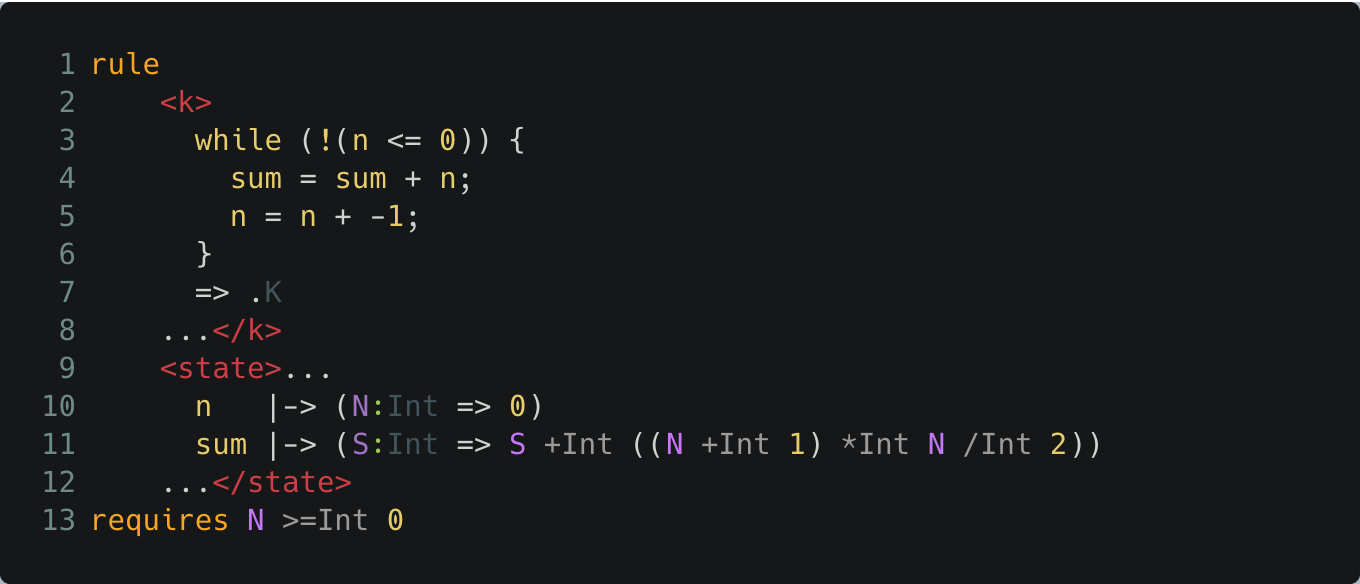The loop invariant in K

The rule specifies the property that, assuming `n` is initially mapped to a non-negative integer value `N` and `sum` is mapped to some integer value `S`, the while loop of SUM reaches a configuration where the loop terminates and the state maps `n` to zero and `sum` is incremented by the summation of values from 1 to `N`.

Although deductive verification is notoriously manual, requiring normally considerable human effort, K automates a sizable fragment of reachability logic that in practice helps in reducing the manual effort needed in the process. When verifying a property, K takes the semantics specification L of the language and the specification P of the program and regards that as a system of (one-path) reachability rules, and then proves (or disproves) that the system entails the reachability rule `p /\ c => p` specifying the correctness property S. The generated K prover uses the semantics for symbolic execution, reasons internally about patterns and uses an external SMT solver (Z3) to check satisfiability of generated constraints.

In particular, for SUM, assuming that the language specification is given in imp.k, one first compiles the specification using `kompile` as follows (see the Makefile for more compiler options):The K compiler generates K language tools for IMP, including the K prover for IMP. Assuming the SUM program is saved in a file sum.imp and that the rules defining the properties (the loop invariant and the SUM correctness property) are saved in a file sum-spec.k, we may invoke the generated K prover for IMP on SUM and the properties using the command: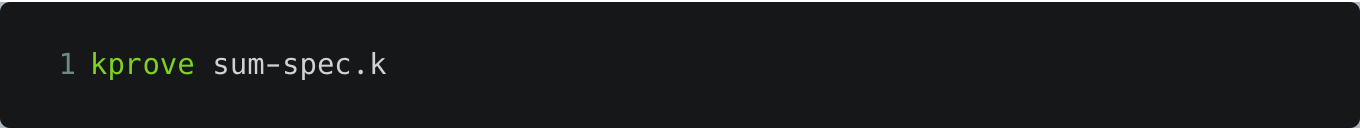The prover automatically verifies the loop invariant and the correctness property of SUM, and indicates that by returning `#True`.

In the example’s repository, we provide a Make file script that automates steps for compiling the specification, running the SUM program and verifying its property. To get a feeling of how efficient verification is with `kprove`, the script also displays the time it takes to complete the task. Within this directory, running the command: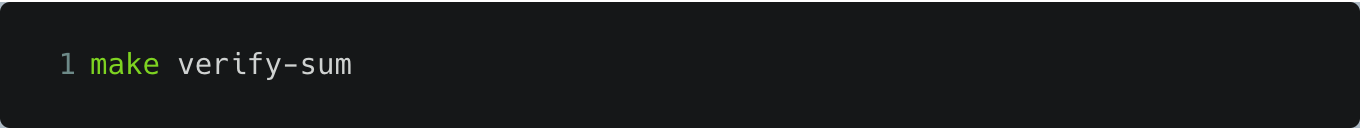runs the verification task (after compiling the specification if needed) and shows the time taken to verify the property. On a 2.9GHz Intel Core i9 MacBook Pro with 32GB of RAM running Ubuntu 18.04.3 LTS on a VirtualBox virtual machine, execution of `kprove`’s verification task takes about 8 seconds to complete.

### Verification in Coq

Following the language-independent coinductive verification approach described here, a program is shown to meet its specification by: (1) using the step relation defining the semantics of the language in which the program is written as a symbolic execution engine, and (2) using simple co-inductive reasoning on circular behaviors emanating from recursive or iterative constructs in the language (such as loops in IMP). The machinery specified in Coq allowing the reachability logic proof strategy can be found in the example’s repository.

As for K above, to prove the correctness property of SUM, we first need to specify and show the loop invariant. For that, we introduce another constructor of the type `sum_spec`: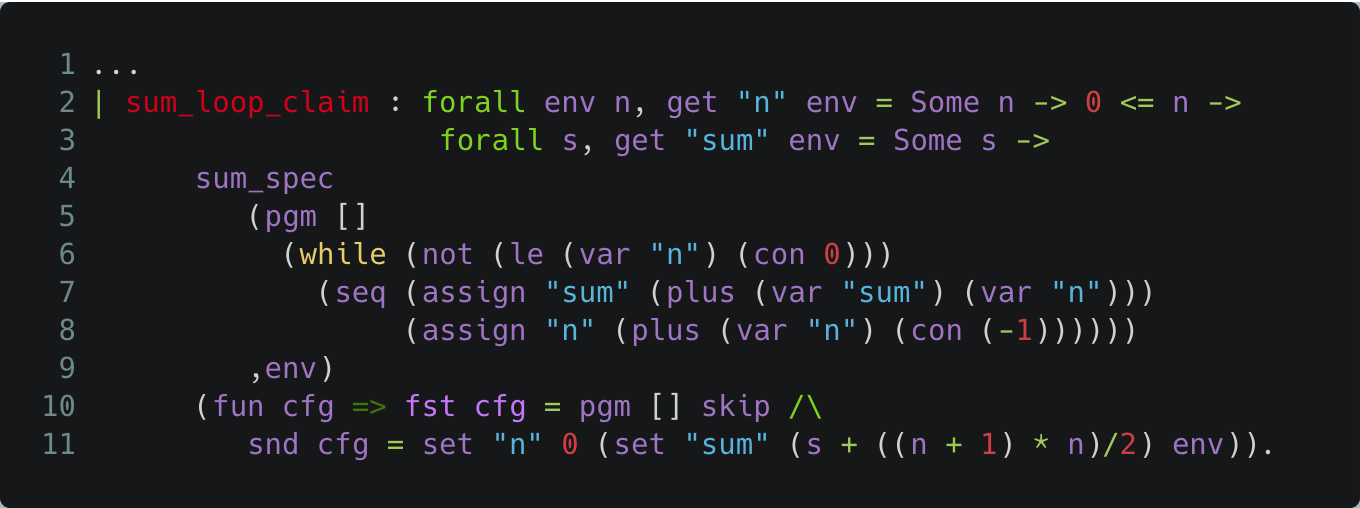The loop invariant in Coq

Note that `get x env` evaluates to the value to which x is mapped in `env` (if defined), while `fst` and `snd` return respectively the first and second components of a state pair. The specification `sum_loop_claim` defines exactly the same loop invariant described above for the K example.

Now, to verify that SUM meets its specification given by `sum_spec`, which is that the initial state of SUM either diverges or terminates with the correct value for `sum`, we instantiate the general coinductive soundness theorem with the step relation `step_p` defining the semantics of IMP, and show the following statement:The correctness lemma of SUM in Coq

`sound` is a generic proposition on reachability claims. The proof of this instantiation is based on a generalized co-induction theorem that mechanizes the verification approach, and is available here.

In comparison with K, the process of checking the reachability claim of the correctness property for SUM in Coq takes a similar amount of time to complete (about 8 seconds) on the same machine. In the example’s repository, we provide a Make script that enables compiling the Coq specification and timing the process of checking the proofs, which can be invoked by the command: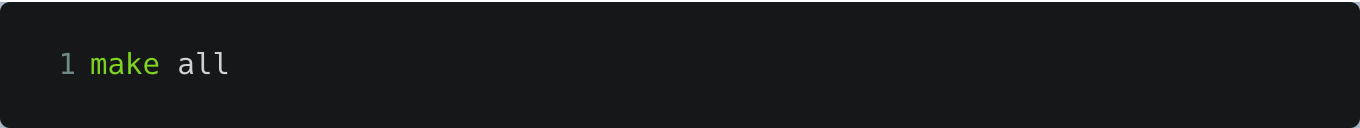## Summary

Both Coq and K are very capable as language verification frameworks and we at Runtime Verification have been using them regularly to provide our formal modeling and verification services. Although our approach enables language-independent program verification in Coq with support for automation, we believe that K is better suited for the task of specifying languages and verifying programs, primarily for the following reasons:

• K was designed from the very beginning to provide a definitional framework for languages in which all program analysis and verification tools for a language are derived directly from its formal specification in the framework. Consequently, K provides several features, not present in Coq, that are specifically tailored for formalizing and analyzing languages, including:

• Support for defining a language’s syntactic structures using the standard notation of BNF along with several syntactic and semantic annotations that enable concise yet precise definitions (vs. inductive purely syntactic definitions in Coq).
• Support for generating language parsers automatically from the BNF specification, so a program’s structure is defined by its text (vs. syntactic program encodings in Coq).
• Support for defining complex operational behaviors using computational structures and continuations, nested-cell configurations, pattern matching and configuration abstraction (vs. low-level set-theoretic denotations in Coq).
• K’s specification is executable, and thus a specification of a language’s syntax and semantics yields immediately an interpreter for the language with which programs can be simulated and tested. Specifications in Coq are generally not executable, although some degree of automation of proofs is achievable.

• By rewriting over patterns with variables, a specification of a language can also be used as a symbolic execution engine for the language. Coupled with other advanced techniques (such as unification, constraint solving and co-inductive reasoning), the symbolic engine can be used for verifying reachability claims in Reachability logic.

Coq, on the other hand, provides a very general framework for modeling any mathematical domain and proving arbitrarily complex mathematical theorems, but does not commit to any specific modeling style beyond the basic principles of type theory and inductive constructions. This obviously results in a more flexible system, but as a language verification framework, and especially when compared with K, this flexibility can be costly (in terms of both effort and time) when formalizing a language and verifying programs. We believe that K strikes a good balance as a language verification framework in which languages can be efficiently formally modeled and verified while maintaining a high level of generality and flexibility. It is worth mentioning that there are current efforts to extend K into a fully fledged proof assistant for matching logic, which will offer the same level of flexibility that Coq offers.

We conclude by noting that both Coq and K are being actively developed and have quite large communities behind them (see for example the projects’ repositories for K and Coq). Furthermore, many of the highlights in this post series come from active areas of research in formal methods and program verification.